# PSAT Math : Statistics

## Example Questions

### Example Question #31 : How To Find Arithmetic Mean

The test average for a class of 13 students is 87%. If two students take make-up tests the next day and score 97% and 56% respectively, what is the new average?

The average does not change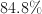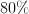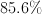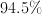Explanation:

In order to calculate the new average, we can act as though each of the thirteen students who originally took the test each earned 87%: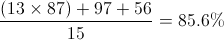### Example Question #32 : How To Find Arithmetic Mean

Tom makes a bet with his friend Bob.  Bob wagers $100, but Tom decides to make him a deal. If Bob wins, Tom will pay him$100.  If Tom wins, Bob will instead pay $1 for the first day, then every day for 10 days he must match the total amount paid so far. For the first day, Bob will pay$1.  The second day, he will pay an additional dollar, since he has only paid $1 thus far. The third day he will pay$2, the fourth $4, and so on. If Bob has a 75% chance to win, which of the following is the closest to the amount of money Tom will make on average with this bet? Possible Answers: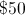-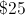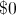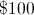Correct answer:Explanation: We are asked to find out how much Tom would win on average. Since Bob has a 75% chance to win, Tom must have a 25% chance to win. The amount Tom will lose is given to us:$100.  We now need to find how much he will win.

The first day, Bob will only pay $1. Similarly for the second day. The third day Bob will now have to pay$2.  The fourth day, $4. We notice that each day we will be doubling how much he pays (since he is matching how much was paid previously). Thus he is paying: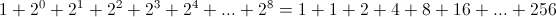Now, there are a couple ways to deal with this series. There are only 10 terms, so we can simply add them up to get our answer. Another method would be looking at it as a geometric series. If you recall, the sum of a geometric series (series that have terms differentiated by a constant multiplier) is given by: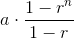whereis the initial value,is the ratio between any 2 terms, andis the number of terms. Here we just have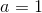, since the first term is a 1. Each term is half the previous term, so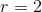, and we have 9 terms (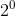to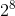) so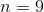. Hence this would be: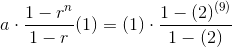and our answer would be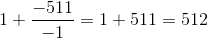We now have that Bob will have to pay$512 if Tom wins, while Tom will have to pay $100 if Bob wins. We finally take into account the odds of winning. 25% of the time, Tom will win$512.  75% of the time he will lose $100. We can more easily interpret this with fractions: 1/4 chance to win, 3/4 chance to lose. Now we simply keep in mind how averages work: The numerator is going to be the sum of all the cases, while the denominator is the number of cases. In this situation, we have 4 cases. 3 of them Tom loses, 1 he wins. Thus we have: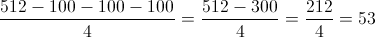So on average, Tom will make$53 with this bet.  The closest answer to this is \$50.

### Example Question #41 : Statistics

Choose the statement(s) that holds true for the following set of data:

{50, 63, 54, 59, 67, 61, 54, 68, 58, 66}

I. The median is 60.

II. The mean is 60.

III. There is no mode.

I and II are true.

II is only true.

I and III are true.

I is only true.

I, II, and III are true.

I and II are true.

Explanation:

List the numbers in order to find the median:  {50, 54, 54, 58, 59, 61, 63, 66, 67, 68}.  The middle numbers are 59 and 61 so their average is 60 for the median of the data set making statement I true.  Add the list of numbers and divide by 10 to get a mean of 60, making statement II true.  There is a mode of 54 so statement III is false.

### Example Question #41 : How To Find Arithmetic Mean

Jim drives for 1 hour at 33 miles per hour, 3 hours at 45 mph and travels at 60 mph for 30 minutes.  What is his average speed during the trip?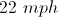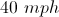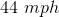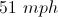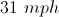Explanation:

To find the average speed, add up the total distance traveled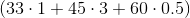and divide by the total time (4.5 hours).

### Example Question #41 : Data Analysis

Susie drove 100 miles in 2 hours. She then traveled 40 miles per hour for the next hour, at which point she reached her destination. What was her average speed for the entire trip?

50 miles per hour

47 miles per hour

48 miles per hour

45 miles per hour

43 miles per hour

47 miles per hour

Explanation:

Distance = Rate * Time

We are solving for the rate. Susie was driving for a total of 3 hours. The distance she traveled was 100 miles in the first leg, plus 40 miles (40 miles per hour for one hour) in the second leg, or 140 miles total. Use the total distance and total time to solve for the rate.

140/3 = 46 2/3 miles per hour (roughly 47 miles per hour)

### Example Question #42 : Data Analysis

If the average (arithmetic mean) of,, and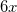is twelve, what is the value of?Explanation:

The mean will be equal to the sum of the given values, divided by the number of given values.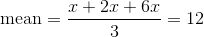Use this equation to solve for.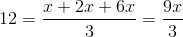Multiply both sides by 3.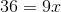Divide both sides by 9.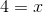### Example Question #41 : Statistics

On Monday the temperature is 58 degrees, on Tuesday it is 64 degrees, and on Wednesday it is 70 degrees. On Thursday, it is five degrees hotter than it was on Monday. Friday, Saturday, and Sunday are all the same temperature as Tuesday. What is the average temperature for the week, rounded to the nearest whole degree?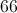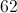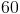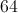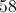Explanation:

Thursday is 5 degrees hotter than Monday, so on Thursday it is: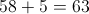Friday, Saturday, and Sunday are the same temperature as Tuesday, so they are all 64 degrees. Now we know all the necessary information to calculate the average.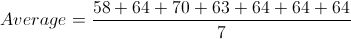The best answer choice is therefore 64.

### Example Question #42 : Statistics

What is the arithmetic mean of all of the odd numbers between 7 and 21, inclusive?Explanation:

One can simply add all the odd numbers from 7 to 21 and divide by the number of odd numbers there are. Or, moreover, one can see that 14 is halfway between 7 and 21.

### Example Question #47 : Statistics

The average age of a certain group of 20 people is 25 years old. Another group of 10 people with an average age of 40 years comes in and joins the first group. What is the average age of the new group?

25

35

32.5

40

30

30

Explanation:

We cannot just take the average of the ages 25 and 40, which is 32.5 years old.

Instead, we need to take a weighted average, taking into account the varying number of people in each group.

Take the average age of each group and multiply it by the number of people in that group and then take the sum. Next divide by the total number of people to get the weighted average age of the new group.

(20 * 25 + 10 * 40)/30  =  30

### Example Question #42 : How To Find Arithmetic Mean

In Stacey's history class, her overall class grade is the arithmetic mean of 5 exams. If her average on the first 4 tests is 85, and she scores 100 on her last exam, what will her final grade in the class be?

100

92

88

84

96

The arithmetic mean can be though of as (total number of points)/(total number of tests). The total number of points that Stacey earned is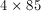for the first 4 tests, plus 100 for the final test.
Stacey's final grade is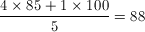.